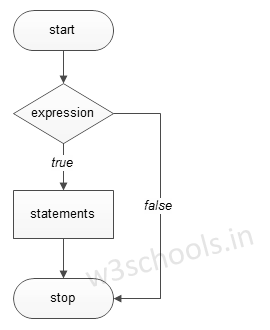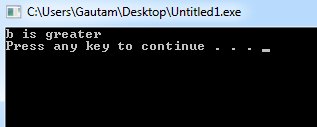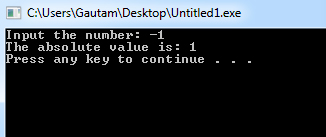## C++ Programming Tutorial Index

C++ `if` Statements control the flow of the program based on conditions. If the expression evaluates to `true`, it executes certain statements within the if block; Otherwise, execution will get skipped. It is the simplest way to modify the control flow in a C++ program. This tutorial will teach you how to use `if` Statements in C++.

Programmers can use "if Statements" in C++ in various forms depending on the situation and code complexity.

There are four different types of if statements in C++. These are:
• if Statement
• if-else Statement
• Nested if-else Statement

The basic format of the `if` Statement is:

Syntax:

``````if(test_expression)
{
Statement 1;
Statement 2;
...
}``````

'Statement n' can be a statement or a set of statements, and if the test expression evaluates to `true`, the statement block will get executed or skipped.

Figure - Flowchart of `if` Statement:

###Example of a C++ Program to Demonstrate `if` Statement:

Example:

``````#include <iostream>
using namespace std;

int main()
{
int a = 15, b = 20;

if (b > a) {
cout << "b is greater" << endl;
}
system("PAUSE");
}``````

Program Output:Example:

``````#include <iostream>
using namespace std;

int main()
{
int number;

cout << "Input the number: ";  cin >> number;

/* check whether the number is negative number */
if(number < 0)
{
/* If it is a negative then convert it into positive. */
number = -number;
cout<<"The absolute value is: "<< number<<endl;
system("PAUSE");
}
} ``````

Program Output: